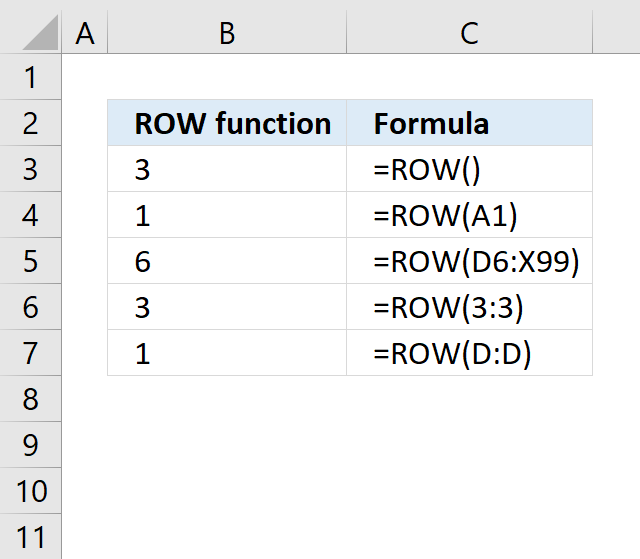Author: Oscar Cronquist Article last updated on May 02, 2018The ROW function calculates the row number of a cell reference.

ROW(reference)

Arguments

 reference Optional. A reference to a cell you want to know the row number of.

You can enter a reference to a single cell or a cell range. The ROW function returns an array of numbers if you enter a reference to a cell range. Remember to enter the formula as an array formula.

The reference argument is optional. Enter =ROW() in a cell and a reference to the cell itself is used. Example, =ROW() in cell F4 returns 4.

The first example demonstrates basic usage. Example 2 and 3 are more advanced and requires some knowledge about MATCH and INDEX function.

Example 1A cell reference can be relative or absolute or a combination of relative and absolute.

Absolute and relative references in excel

In cell B2: =ROW(B2) returns 2.

In cell B6: =ROW() returns 6

In cell B11: =ROW(\$D\$10) returns 10 (absolute cell reference).

Example 2 - Duplicate columns in an arrayIn cell range B3:B5:

=ROW(\$C\$3:\$D\$5)

returns {3; 4; 5}

Now you might wonder why the array formula doesn´t return {3, 3; 4, 4; 5, 5}. There are six cells in the cell range, how come only three values are returned?

The answer is that there is no need for multiple duplicate columns in the array. Excel simplifies the array down to a single column. But when used with multiple cell ranges in more complicated array formulas, make sure the number of rows match. See this example: Unique distinct values from a cell range

Example 3 - Row numbering a cell rangeThe INDEX function returns a value of the cell at the intersection of a particular row and column, in a given range. To be able to work with multiple values from an arbitrary cell range using the INDEX function we must number each row. That is exactly what we did in the previous example but the first row in a given range has to be 1 and the second 2 and so on. Why? The INDEX function needs properly numbered cell ranges.

This is where the ROW and MATCH function comes in.

=MATCH(ROW(cell_range), ROW(cell_range))

The beauty with this formula is that it row numbers any cell range with any size.

In this example, the cell range is C3:D5:

=MATCH(ROW(C3:D5), ROW(C3:D5))

becomes =MATCH({3; 4; 5}, {3; 4; 5}) and returns {1; 2; 3}.

Remember, enter the formula as an array formula.

So what can you do with row numbered cell ranges?

The MATCH function can also be used to find the relative position of a value in a cell range. See these posts: